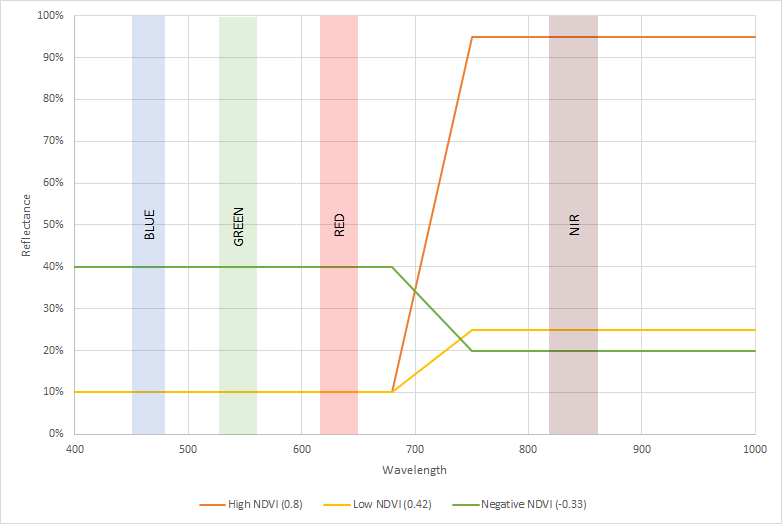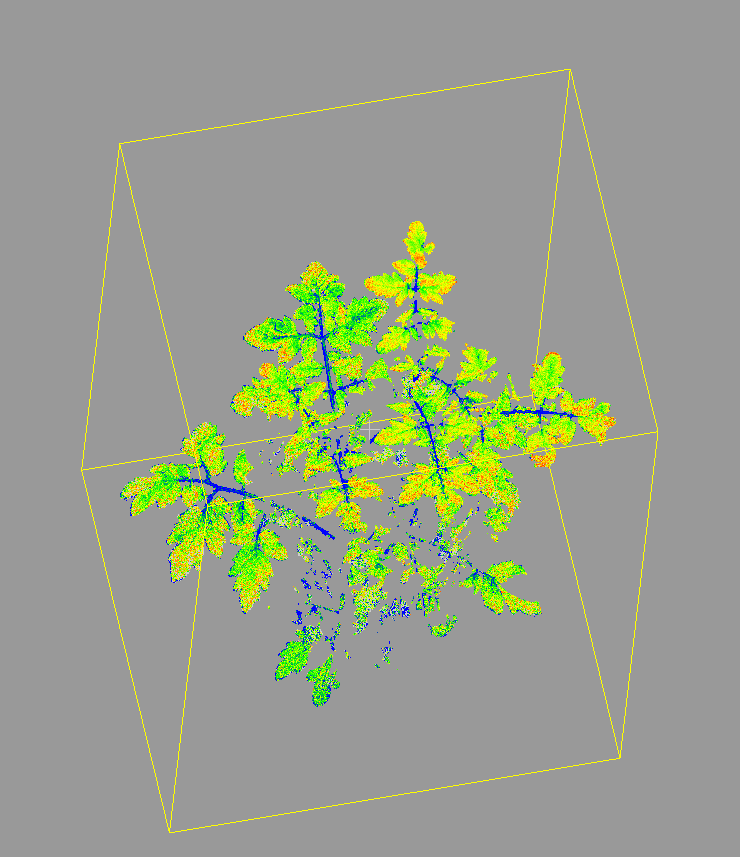Value Range: -1–  1

• Normalized differential vegetation index
• (NIR – RED)/(NIR + RED)
• Correlated to PAR interception

NDVI is computed as the ratio of the NIR and the Red channel in PlantEye. It is computed as the difference of both channels divided by the sum of both channels. Therefore it is a ratio in the range of -1 to 1. The illustration below shows three different examples for the calculation of NDVI. The X axis represents a simplified spectrum in the range from 400 to 1,000nm. The Y axis shows the relative reflectance for each wavelength. The colored blocks represent the measurement channels of PlantEye with standard configuration.One can see that there are three different examples that show the value for the NDVI index. The first example shows a high NDVI where the reflectance in the NIR channel is much higher than in the Red channel. This is the typical effect for healthy vegetation. The index is around 0.8. The second example shows a low NDVI. In this case the reflectance in the NIR channel is only slightly bigger than in the Red channel. This is a typical pattern for unhealthy vegetation. The last example shows that in theory the NDVI value could also be negative if the Red reflectance is higher than the NIR reflectance. This is typically not observed in any plant related measurements.